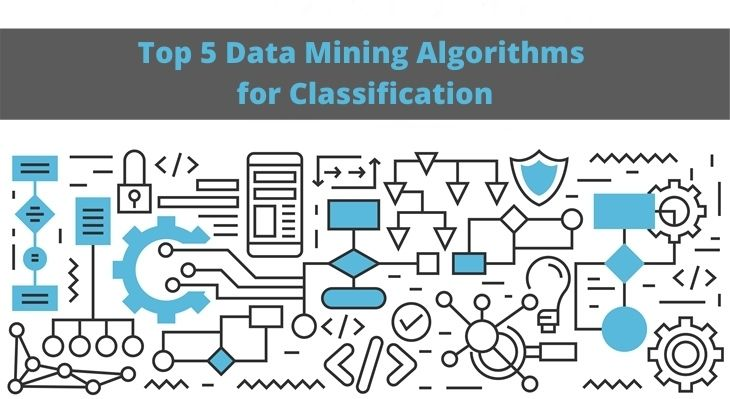## Top 5 Data Mining Algorithms for Classification

Classification is an expanding field of research, particularly in the relatively recent context of data mining. Classification uses

# Top 5 Data Mining Algorithms for Classification

Classification is an expanding field of research, particularly in the relatively recent context of data mining.

Classification uses a decision to classify data. Each decision is established on a query related to one of the input variables. Based on the acknowledgments, the data instance is classified.

A few well-characterized classes generally provide an efficient synthesis of the set of objects. As such, a classification is a powerful tool for data exploration.

In this article, we’ll go through what data mining is and explore the best data mining algorithms for data mining.

## What Exactly is Classification?

Classification is one of the most commonly used technique when it comes to classifying large sets of data.

This method of data analysis includes algorithms for supervised learning adapted to the data quality.

The objective is to learn the relation which links a variable of interest, of qualitative type, to the other observed variables, possibly for the purpose of prediction.

The algorithm that performs the classification is the classifier while the observations are the instances.

Classification is used when the variable of interest is qualitative.

The classification method uses algorithms such as decision tree to obtain useful information. Companies use this approach to learn about the behavior and preferences of their customers.

With classification, you can distinguish between data that is useful to your goal and data that is not relevant.

An example of this would be your own email service, which can identify spam and important messages.

## Top 5 Data Mining Algorithms for Classification

### Decision Trees

Decision trees are considered to be the latest in data mining algorithms.

They help to analyze which parts of the database are really useful or which part contains a solution to your problem.

It is a support tool that uses a decision chart or model and its possible consequences. That includes results of chance events, resource costs, and utility.

From a decision perspective, a decision tree is the least number of questions that must be acknowledged to evaluate the likelihood of making an accurate decision.

By looking at the predictors or values ​​for each split in the tree, you can draw some ideas or find answers to the questions you have asked.

Decision trees enable you to approach the obstacle in a structured and systematic behavior.

### Logistic Regression

Logistic regression is a statistical method of creating a binomial result with one or more descriptive variables.

This algorithm aims to determine whether an instance of a variable falls into a category.

Usually, regressions can be used in applications, such as:

• Credit score
• Measure the success rates of marketing campaigns
• Predict revenue for a particular product

### Naive Bayes Classification

Naive Bayes is a simple classification algorithm, which uses historical data to predict the classification of new data.

It calculates the probability that an event will occur given that another event has already occurred.

They allow us to predict the likelihood of an event occurring based on conditions that we know for the events in question.

Some real-life examples of Naive Bayes classification are:

• To filter an email as spam or not-spam
• Rate a news article about technology, politics or sports
• Used for facial recognition software

### k-nearest neighbors

The k-nearest neighbor method is a standard classification algorithm that is based exclusively on the choice of the classification metric.

First, we use a set of data to train the algorithm. Subsequently, the distance between the training data and the new data is evaluated to categorize the new data.

Depending on the size of the training set, this algorithm can be computationally intensive. To make a prediction, the K-NN algorithm will be based on the entire data set.

The K-NN algorithm assumes that similar objects exist nearby. In other words, similar items are close to each other.

They receive unclassified data and measure the distance of the new data in relation to each of the other data that are already classified.

Yet, it is often used for its simplicity of use, ease of training, and the ease of interpreting the results.

### Support Vector Machine

Support Vector Machine (SVM) is a supervised machine learning algorithm that can be used for classification, regression, and anomaly detection purposes.

SVMs are based on the idea of ​​finding a hyperplane that best divides a dataset into two classes.

The SVM algorithm aims to find the separation between two object classes with the idea that the larger the separation, the more robust is the classification.

Some of the biggest problems that have been solved using SVM are: